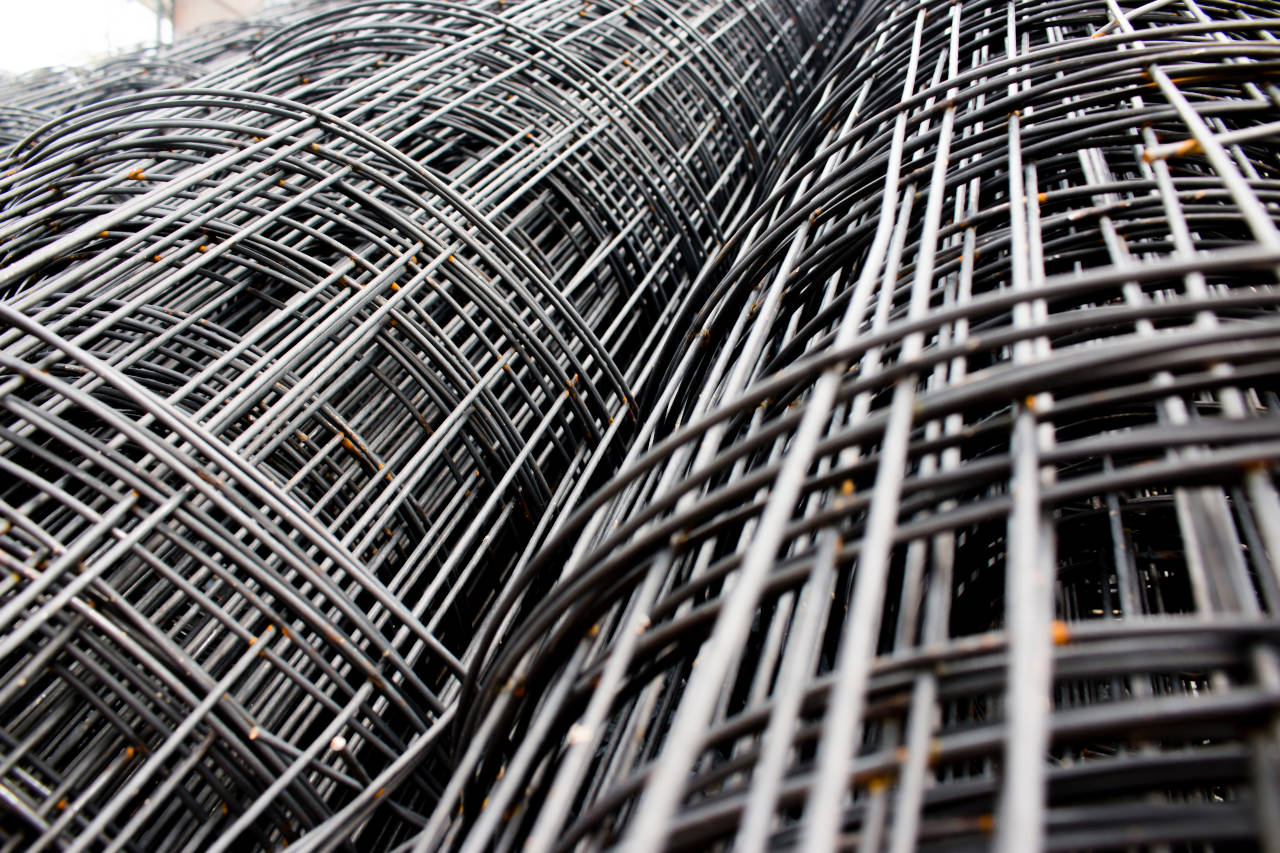# Concrete Reinforcing Mesh Calculator

Enter the dimensions of the slab and the size mesh used to calculate the amount of concrete reinforcement needed.

## Calculate using Slab Length & Width

Slab Size
Mesh Roll / Sheet Size
%
Optionally enter the price per roll/sheet
\$

## Calculate using Slab Area

Slab Size
Mesh Sheet/Roll Size
%
Optionally enter the price per roll/sheet
\$

## Results:

rolls / sheets
\$
estimated Cost
Learn how we calculated this below

## How to Estimate Concrete Reinforcement Mesh

Mesh is a popular material used to reinforce concrete slabs and pours. It may be used in place of rebar for thinner slabs or in addition to rebar for thicker pours.

Mesh may be wire or made of fiber. Metal mesh is laid before the concrete is poured, then lifted to the center, while fiber mesh is placed in the concrete evenly as it is poured.

Both these materials are common for projects like sidewalks and patios that may not hold heavy loads. The amount of mesh reinforcement needed for a project can be calculated in a few easy steps.### Step One: Calculate the Slab Area

The first step to estimating reinforcement is to find the size of the concrete slab that will be poured. If you already know this figure, then you can skip ahead to the second step.

The area of the pour can be found by multiplying the length in feet by the width in feet. The result is the square footage of the slab.

Here’s the formula to find area:

area = length × width

For shapes other than a rectangle, you’ll need the appropriate formula to calculate the area, or you can use a square footage calculator.

### Step Two: Calculate Mesh Coverage

The second step is to find the coverage of the mesh roll or sheets that will be used for the project. Sometimes the coverage in square feet is provided by the manufacturer, but if not, it can be found using the area formula above.

### Step Three: Divide Slab Area by Reinforcement Area

The final step in estimating the number of rolls or sheets of mesh reinforcement is to divide the square footage of the slab by the square footage of the reinforcing mesh. This is the formula:

mesh needed = slab area ÷ mesh area

For example, let’s find the number of 5′ x 50′ rolls of mesh required to reinforce a slab that is 25′ by 25′.

First, find the area of the slab.

area = 25′ × 25′
area = 625 sq ft

Next, find the coverage of the roll.

coverage = 5′ × 50′
coverage = 250 sq ft

Finally, divide the slab area by the coverage.

rolls needed = 625 sq ft ÷ 250 sq ft
rolls needed = 2.5

Thus 2 ½ 5′ x 50′ rolls will be needed to reinforce a 25′ x 25′ slab.

## How to Account for Lapping

One thing to consider when calculating reinforcing mesh material is to account for overlap when using wire mesh. Lapping is required with wire mesh to improve the strength around the edge of the mesh and resist cracking.

Fiber mesh does not require any overlap because of the way that it is distributed through the concrete during the pour.

Wire mesh should overlap at all edges and ends by a minimum of 2 ½”. Larger overlaps are fine and sometimes necessary if the material is having difficulty laying flat.

To account for lapping, calculate the overlap as a percentage of additional material needed. The formula to find the overlap percentage is:

lapping percentage = overlap ÷ reinforcement width

For instance, let’s find the lapping percentage for a 6″ lap when using a 5′ wide roll of mesh. Note that 5′ is equal to 60″, which is the figure used in the formula below.

lapping percentage = 6″ ÷ 60″
lapping percentage = .10

Thus, a 6″ lap will require 10% additional material because .10 is equal to 10%.

Don’t forget to try our concrete calculator to determine the right amount of concrete needed for your next pour.